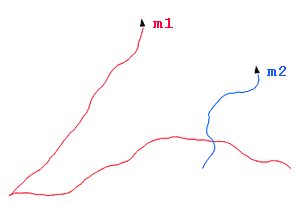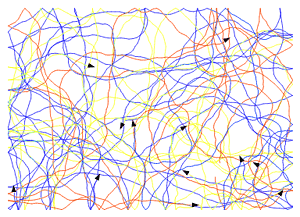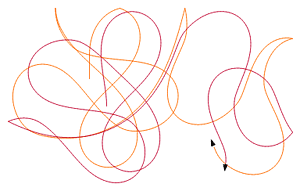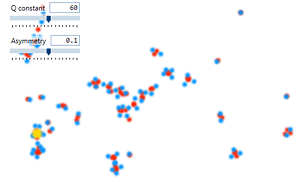## Simulations and models

In this chapter we will create a simulation of the behaviour of many interacting objects. A class describing the behavior and properties of these objects will be defined. We will show how to control them with the use of a timer and signals, and how to pass variable values between the objects.

This chapter is dedicated to intermediate-level users. Before reading, it is advisable to look into the handbook, into the chapter on POOL classes.

### 1. The first model.At the beginning, we will write a very simple class, to be developed in following chapters.
A class in POOL is defined exactly in the same way as a function. In fact, a new object (a turtle) performs at the creation stage the function that defines its class. All the functions and variables then created become the members of that object. Also the parameters of the creating function are preserved for the object’s lifetime as local variables.

In the first version of the program, a `model` class allows for setting simple features of a turtle: its initial position, heading and speed. These values are passed as arguments of the function that creates the turtle - let’s call this function the constructor. The constructor sets also a drawing colour and a radius of the turtle (now used at bouncing off a wall). Finally, the constructor outputs information on the turtle’s new parameters in a text window.
A `model` class has one member function: `step dt`. This function allows to move a turtle forward by a distance resulting from its speed (given as the constructor’s argument) and the `dt` period of time given as an argument of the function.

The main turtle (`#first`) program creates two `model` class turtles. The description of the `newturtle` command used to do this can be found in the handbook. To move the turtles, the `repeat` loop calls the `step` function for each of them. The function to be performed by objects is called by the `@` operator in POOL.
A delay in a loop allows to keep a constant pace of program operation execution, appropriate for turtle speed settings.

;turtle constructor with arguments:
; p - initial position {x y}
; h - initial direction in degrees
; v - speed in pixels / second
to model :p :h [:v 60]
setpos :p
setpencolor random 1000
pendown
showturtle

;member function that moves turtle forward,
;step size depends on variable v of the turtle and
;time in milliseconds, passed to the function as
;the argument dt
to step :dt
right (runif -20 20)     ;random turn
forward 0.001 * :dt * :v ;step forward
end

(print who "|p =| :p "|h =| :h "|v =| :v)
end

;configuration:
; -turtles will bounce off a wall
bounce
; -turtle #first is invisible and does not draw lines
hideturtle penup

;two turtles of the "model" class, ready for commands:
"m1 := (newturtle \$model {-50 0} 30 15) ;left, a bit slower
"m2 := (newturtle \$model {50 0} (-50))  ;right, default speed

;step every 0.1s - you may try different values
"dt := 100
repeat 500 [
sync :dt ;delay
(step :dt) @ :m1
(step :dt) @ :m2
]

### 2. More independence.In the previous chapter, the `#first` turtle controlled the `:m1` and `:m2` turtles, by issuing commands for them in a loop. A better way of regular command calling is to do it with a timer. A timer does not require programming of delays (`sync` or `wait`) and does not block the access to the `#first` turtle in the command line. In the next version of the program we will use a timer.
The handbook explains how to use a timer. Now, we will use a version, where a timer sends a signal to all the turtles at regular intervals. In the class describing our model, we can receive such a signal with an appropriate handling function - such function will replace the `step` function from the previous chapter. A value of time needed to calculate the length of the turtle step can be taken from the information included in the signal sent by the timer.

At this stage, we also change the method a new turtle is created. An event handling function is added to the `#first` turtle program - it handles clicking a mouse (see the description in the handbook). At each click, a new turtle will now appear in the graphic window, and it will immediately begin to handle the signals sent by the timer.

After starting the program and creating a few turtles, switch into the command line. You can give commands - the `#first` turtle is busy with executing the timer function for a mere fraction of a millisecond. You can check e.g. how many turtles have been created so far: `print count children`.

;turtle constructor
to model :p :h [:v 60]
setpos :p
(setpencolor random 1000 75)
pendown
showturtle

;handling funkction for the "step" signal
to onsignalstep :turtle :data
right (runif -20 20)
let "dt :data,2 ;time from the previous iteration of timer
forward 0.001 * :dt * :v
end

(print who "|p =| :p "|h =| :h "|v =| :v)
end

;create new turtle on each click
to onmouseclick :mousepos
let "m (newt \$model :mousepos random 360 20 + random 100)
end

;configuration of the #first turtle
bounce ht pu

;send signal "step" every 0.5sto all turtles,
;try higher frequency of the timer:
;use values: 100, 50, 20
"t := timer "step 500

### 3. More cooperation.In previous programs, each turtle followed its own track and there was no interaction between them. In this chapter, we will make them communicate. Instead of executing random turns, we will calculate a new direction of a turtle in each step - slightly towards other turtles.
The `onsignalkrokstep` handling function is changed - instead of the `right (runif -20 20)` turn, we will use a command that will position a turtle according to a given vector: `setheaddir turn`; `turn` is a new member function of the `model` class. It includes a several new elements:

`all` - a command returning the list of all the turtles existing in the program;

`this` - a command returning the turtle which called the command; in this case, it allows to avoid calculating the "towards itself" direction: `:m <> this`;

`pos @ :m` - reading the position of other turtles.

;turtle constructor
to model :p :h [:v 60]
setpos :p
(setpencolor random 1000 75)
pendown
showturtle

;function to calculate new direction
to turn
;only if there are at least 3 turtles
if (count all) > 2 [
let "sx 0 let "sy 0
foreach "m all [
;do not calculate direction to itself
;and skip the #first turtle
if and (:m <> this) (:m <> #first) [
let "pm pos @ :m ;position of the turtle :m
let "dx :pm,1 - xcor
let "dy :pm,2 - ycor
let "norm sqrt :dx^2 + :dy^2
"sx := :sx + :dx / :norm
"sy := :sy + :dy / :norm
]
]
"sx := 0.1 * :sx + 0.9 * headdir,1
"sy := 0.1 * :sy + 0.9 * headdir,2
output array :sx :sy ;new direction
]
output headdir ;current direction, no changes
end

;handling function for "step" signal
to onsignalstep :turtle :data
setheaddir turn ;turn towards other turtles
fd 0.001 * :data,2 * :v
end

(print who "|p =| :p "|h =| :h "|v =| :v)
end

;create new turtle on each click
to onmouseclick :mousepos
let "m (newt \$model :mousepos random 360 50 + random 100)
end

;configuration of the #first turtle
bounce ht pu
setpalette "hot ;another palette, for fun

;send signal "step" every 0.05s to all turtles
"t := timer "step 50The program above looks reasonably and it operates according to our expectations. However, to prepare a more interesting and complex simulation, we need a stricter order - a division into two stages:

calculating changes - preparation of new values for direction, speed, etc.; the state of all the objects is "frozen" and the objects can read each other’s state;

applying changes - the state of objects changes according to the values calculated at the preceding stage; the objects do not communicate with each other.

Such a division allows for simultaneous calculations at each stage, and yields the results that are independent of the calculation running sequence. In order to realize this algorithm, the operation of the timer has to be adjusted so that at each "tick" it would send two signals: the "turn" signal, on which the handling function in the `model` class will calculate new direction of the turtle on the basis of the arrangement of other turtles; and the "step" signal which will make each turtle turn and move a step ahead. The signals in the new program of the timer are "blocking" signals (see the description in the handbook), that is: execution of commands by the program that has sent a signal is stopped until all the objects finish to handle the signal that has been sent.

Another improvement of the new version is the feature that allows for storing the model class turtles in a shared variable (one copy of a variable is available for all the objects): `shared "turtles`. Thus, searching the turtle list while a new direction is being calculated is a bit easier.

shared "turtles ;list of turtles of class model
"turtles := []

to model :p :h [:v 60]
setpos :p
(setpencolor random 1000 75)
pendown
showturtle

;handling function for "turn" signal
to onsignalturn
if (count :turtles) < 2 [
]
let "sx 0 let "sy 0
foreach "m :turtles [
if :m <> this [
let "pm pos @ :m
let "dx :pm,1 - xcor
let "dy :pm,2 - ycor
let "norm sqrt :dx^2 + :dy^2
"sx := :sx + :dx / :norm
"sy := :sy + :dy / :norm
]
]
"sx := 0.1 * :sx + 0.9 * headdir,1
"sy := 0.1 * :sy + 0.9 * headdir,2
"dir := array :sx :sy
end

;handling function for "step" signal
to onsignalstep :turtle :data
fd 0.001 * :data * :v
end

(print who "|p =| :p "|h =| :h "|v =| :v)
end

;create new turtle on each click
to onmouseclick :mousepos
queue :turtles (newt \$model :mousepos random 360 50 + random 100)
end

;configuration of the #first turtle
bounce ht pu
setpalette "blue2red

;every 0.05s:
"t := timer [
signalw "turn      ;blocking signal "turn"
(signalw "step :dt;blocking signal "step"
] 50

### 4. Full simulation.

We have now all the elements needed for programming a simulation. It will be a particle model with a mass–dependent inertia and an attracting or repealing electric-like charge. Using GUI sliders, you can change an interaction force (the "force" slider) and mass proportions (the "asymmetry" slider).

shared "particles
"particles := []

to model :q :mass [:v 0] [:h 0] [:ft 0.001] [:c 90]
to set_force :g    ;set new value of the interaction constant:
"c := -(:q * :g;charge * interaction constant
end

to set_mass :m  ;set new mass of the particle
;radius depends on the mass value:
setr 10 * sqrt :m
"mass := :m
end

"charge := :q ;publicly accessible value of the charge
set_mass :mass
set_force :c
setpos pos @ parent
seth :h
st

let "fx 0 ;sum of forces in X direction
let "fy 0 ;sum of forces in Y direction
to onsignalf  ;calculate sum of forces on "f" signal
"fx := 0 "fy := 0
foreach "m :particles [
if :m <> this [
let "p pos @ :m
let "d distance :p
ifelse :d > :r
[let "fm (:c * :charge @ :m) / :d^3]
[let "fm (:c * :charge @ :m) / :r^3]
"fx += :fm * (:p,1 - xcor)
"fy += :fm * (:p,2 - ycor)
]
]
end

to onsignalstep :turtle :data
let "dt 0.01 * :data
if :dt > 0.8 ["dt := 0.8]

:hdir,1 := :v * :hdir,1 + :fx * :dt / :mass
:hdir,2 := :v * :hdir,2 + :fy * :dt / :mass
"v := sqrt :hdir,1^2 + :hdir,2^2
if :v > 30 ["v := 30]

let "ds :v * :dt
"v -= :ds * :ft / :mass
if :v < 0 ["v := 0]
fd :ds
end
end

bounce ht pu

repeat 30 [ ;red particles, "positive", heavier
setxy (rnorm -30 30) (rnorm -10 30)
"m := (newt \$model 1 0.7)
(setturtleimg "red_light_small) @ :m
queue :particles :m
]

repeat 30 [ ;blue particles, "negative", lighter
setxy (rnorm 30 30) (rnorm -10 30)
"m := (newt \$model (-1) 0.3)
(setturtleimg "blue_light_small) @ :m
queue :particles :m
]

home
;one yellow, heavy particle:
"m := (newt \$model 3 2 4 45 0)
(setturtleimg "yellow_light) @ :m
queue :particles :m

;slider for "interaction constant"
"gs := (slider "force {10 4} {10 100 5} 90)
setonchange :gs [
foreach "m :particles [(set_force getvalue :gs) @ :m]
]

;slider for mass proportions
"sym := (slider "asymmetry {10 60} {-0.9 0.9 0.1} 0.4)
setonchange :sym [
let "s 0.5 * (1 + getvalue :sym)
foreach "m :particles [
if :charge @ :m = 1 [(set_mass :s) @ :m]
if :charge @ :m = -1 [(set_mass 1 - :s) @ :m]
]
]

"t := timer [
signalw "f
(signalw "step :dt)
] 25

### 5. Summary.This article presents the most fundamental techniques used for preparing a simulation consisting of many interacting objects: the description of the properties and behavior of objects by classes, the application of a timer for simulation steps to be taken at regular intervals, and signals - to trigger off parallel tasks.
The simulation we prepared is based on very simple principles - inertia and a force resembling an electrostatic force. Even such a simple description allows to notice an interesting behavior of a group of objects. The program can serve as a basis for creating a more realistic description, e.g. complemented with other ways of interaction or with different object models. Interactive elements responding to user’s actions are also worth adding.